# Math in Focus Grade 2 Chapter 5 Practice 4 Answer Key Real-World Problems: Multiplication and Division

Go through the Math in Focus Grade 2 Workbook Answer Key Chapter 5 Practice 4 Real-World Problems: Multiplication and Division to finish your assignments.

## Math in Focus Grade 2 Chapter 5 Practice 4 Answer Key Real-World Problems: Multiplication and Division

Solve.

Question 1.
There are 2 plates. Each plate has 5 strawberries. How many strawberries are there?
5 + 5 = ___
2 × 5 = ___
There are __ strawberries.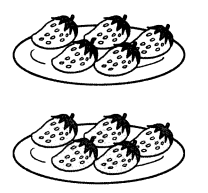Given that
There are 2 plates each plate as 5 strawberries.
To find the number of strawberries there are 2 possible ways they are
5 + 5 = 10
5 × 2 = 10
Therefore there are 10 strawberries.

Question 2.
There are 6 bags. Each bag has 3 pockets. How many pockets are there?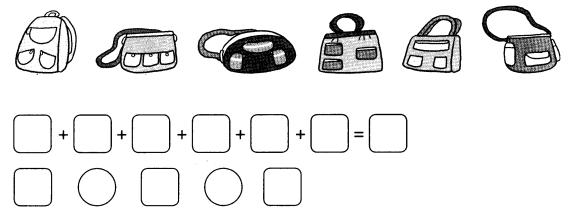There are ___ pockets.
Given that
Number of bags are 6.
Each bag as 3 pockets.
To find the number of pockets there are two possible ways they are
3 + 3 + 3 + 3 + 3 + 3 = 18
6 × 3 = 18
Therefore number of pockets are 18.

Question 3.
Tim has 4- jars. There are 6 marbles in each jar. How many marbles does Tim have altogether?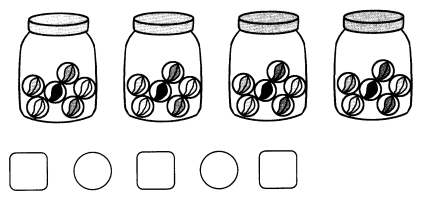Tim has ___ marbles altogether.
Given that
Number of jars near tim = 4
There are 6 marbles in each jar.
Number of marbles = 6 × 4 = 24.
Tim has 24 marbles altogether.

Question 4.
Yanthi has 5 boxes. There are 8 pencils in each box. How many pencils does she have altogether?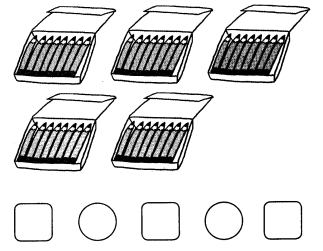Yanthi has ___ pencils altogether.
Given that
Yanthi has 5 boxes.
There are 8 pencils in each box.
Number of pencils = 8 × 5 = 40
Yanthi has 40 pencils altogether.

Question 5.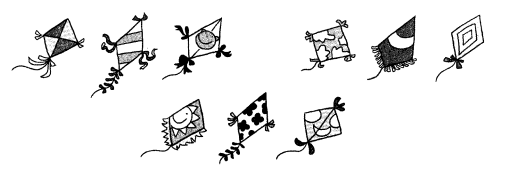Aunt Emma has 3 nephews. She buys 9 kites. She gives each nephew an equal number of kites. How many kites does each nephew get?
____ – ___ – ___ – ___ = 0
______ ÷ ___ = ____
Each nephew gets __ kites.
Given that
The Aunt Emma has 3 nephews
She gives each nephew an equal number of kites.
9 – 3 – 3 – 3 = 0
9 ÷ 3 = 3
Each nephew gets 3 kites.

Question 6.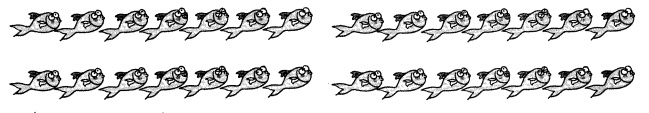Mr. O’Brien catches 28 fish. He puts 7 fish in each bucket. How many buckets does he have?
____ – ___ – ___ – ___ = 0
______ ÷ ___ = ____
He has __ buckets.